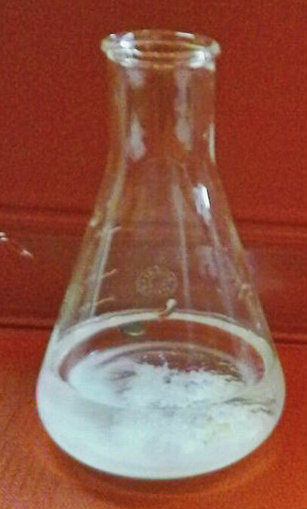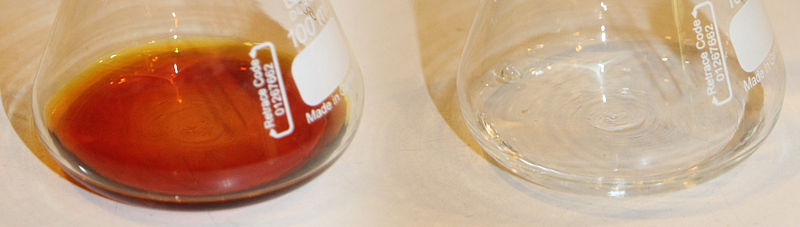# Difference Between Volumetric Analysis and Titration

## Main Difference – Volumetric Analysis vs Titration

There are various chemical techniques to analyse an unknown sample. Some of these techniques are simple whereas other techniques are very advanced. Volumetric analysis is a simple technique that can be used to analyse components of a solution. Since this technique is applied regarding the volumes of compounds, it can only be applied for solutions. Often, volumetric analysis is also called titrimetric analysis or titration. But there is a difference between volumetric analysis and titration when considering their applications. The key difference between volumetric analysis and titration is that the term volumetric analysis is used where analysis is done to analyse a solution for several different unknown values whereas the term titration is used where the concentration of an unknown component of a solution is determined.

### Key Areas Covered

1. What is Volumetric Analysis
– Definition, Technique, Examples
2. What is Titration
– Definition, Technique, Examples
3. What is the Relationship Between Volumetric Analysis and Titration
– Volumetric Analysis and Titration
4. What is the Difference Between Volumetric Analysis and Titration
– Comparison of Key Differences

Key Terms: Molar Mass, Stoichiometry, Titrand, Titrant, Titration, Titrimetric Analysis, Volumetric Analysis## What is Volumetric Analysis

Volumetric analysis is a practical technique whereby one uses reacting volumes to analyse and calculate a variety of unknown values. A volumetric analysis can be used to determine the concentration of a solution, the molar mass of a component in a solution, the percentage of a component present in a solution, formula of a substance or stoichiometry of an equation. When it is used to determine the concentration of a solution, it is called a titration.

If the unknown substance is given in a granular or a powdered form, first it should be dissolved in a suitable solvent in order to obtain a solution. Then this solution can be titrated with a suitable standard solution that has a known concentration. The apparatus used in the volumetric analysis should be free from contamination. Otherwise, there would be different side reactions that are unfavorable.

When measuring volumes, one should use appropriate equipment. Otherwise, the technique will give faulty results. By obtaining an average value by doing the same experiment several times will minimize the errors. One should also choose suitable indicators for the determination of the endpoint of the analysis.Figure 1: A precipitation reaction as an example for the determination of the percentage of a component through volumetric analysis.

Some common applications of volumetric analysis are acid-base reactions, redox reactions, and complexometric reactions. All these reactions involve the analysis of a certain volume of a given unknown sample with a known solution. Sometimes, indicators are not used because reactants can act as self-indicators. By determining the amount of the known compound reacted with the analyte compound, we can determine the concentration, percentage, molar mass and other factors with suitable calculations.

## What is Titration

A titration is a practical technique used to determine the concentration of an unknown solution. Therefore, it is a quantitative method. A titration is the reaction between the titrant and the titrand. The titrant is the substance having a known concentration, and the titrand is the analyte compound. The endpoint of the reaction is determined using an indicator. This indicator gives a color change in the solution when the endpoint has been reached. But sometimes, an indicator is not required because the reactants themselves can act as indicators.

There are several types of titrations that are named according to the reactants, products or the application. Some of them are acid-base titrations, redox titrations, precipitation titrations, EDTA titrations, Iodometric titrations, etc.Figure 2: Color Change in Iodometric Titrations

The typical apparatus of a titration includes the addition of titrant from a burette to the Erlenmeyer flask which contains the titrand or the analyte. The indicator is added to the Erlenmeyer flask. At the end point, the reaction mixture in the flask gives a color change. At that point, the burette reading is obtained. From that reading, the amount of titrant reacted can be determined. Then, using stoichiometric relationships, the amount of analyte present in the sample solution can be determined.

## Relationship Between Volumetric Analysis and Titration

Titration is a type of volumetric analysis. When a volumetric analysis is used to determine the concentration of an unknown component in a given solution, it is called a titration.

## Difference Between Volumetric Analysis and Titration

### Definition

Volumetric Analysis: Volumetric analysis is a practical technique whereby one uses reacting volumes to analyse and calculate a variety of unknown values.

Titrations: A titration is a practical technique used to determine the concentration of an unknown solution.

### Determination of Values

Volumetric Analysis: Volumetric analysis determines several different unknown values regarding the analyte.

Titrations: Titrations determine the concentration of an unknown component in a solution.

### Conclusion

Titration is a type of volumetric analysis. All volumetric analysis methods involve titrations. But the term titration is used when a volumetric analysis is done to determine the concentration of an unknown component in a solution whereas the term volumetric analysis is used to determine several other factors as well. This is the key difference between volumetric analysis and titration.

##### References:

1. “Volumetric Analysis – Procedure & Principles.” Chemistry, Byjus Classes, 13 Sept. 2017, Available here. Accessed 18 Sept. 2017.
2. “Wired Chemist.” Volumetric Analysis, Available here. Accessed 18 Sept. 2017.
3. “Titration.” Wikipedia, Wikimedia Foundation, 17 Sept. 2017, Available here. Accessed 18 Sept. 2017.

##### Image Courtesy:

1. “Beli talog AgCl(srebro hlorida)” By Milana995 – Own work (CC BY-SA 4.0) via Commons Wikimedia
2. “Iodometric titration mixture” By LHcheM – Own work (CC BY-SA 3.0) via Commons Wikimedia